# Data Structures

Computing

## Quiz 15 : Graphs and DigraphsStudy FlashcardsLooking for Programming Homework Help?

## Quiz 15 :Graphs and Digraphs

Question TypeUsing the Huffman code developed in Exercise 7, encode the message "feed a deaf aged hag".
Free
Essay

Algorithm for Huffman:
Input:
Characters : [C 1 ,C 2 ,…………C n ]
Weight : [W 1 ,W 2 ,………W n ]
where W i is the weight of C i
Step 1 :
One-node binary tree list is initiated.
Step 2 :
Repeat the process for n-1 times:
• search for the lowest weight in the list W' and W''and draw two trees T' and T''
• the trees are replaced with binary tree which contains the root value as W' + W''
• the subtree pointers are labeled as 0 and 1 respectively
Step 3 :
The character's code can be obtained by label in the path from root to the current node
Given:
Huffman code developed in "Exercise-7":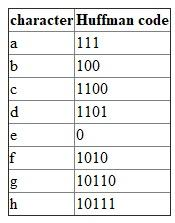Consider the Message: "feed a deaf aged hag":
The Huffman code for the value "f" is as follows: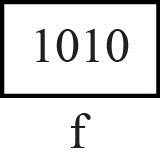The Huffman code for the value "e" is as follows: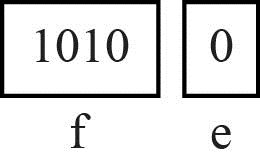The Huffman code for the value "e" is as follows: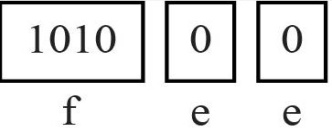The Huffman code for the value "d" is as follows: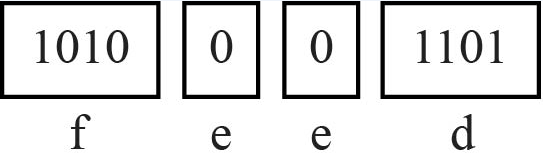The Huffman code for the value "a" is as follows: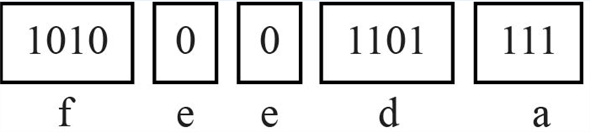The Huffman code for the value "d" is as follows: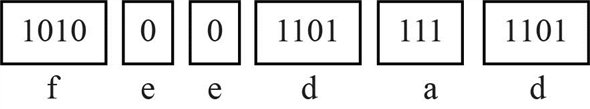The Huffman code for the value "e" is as follows: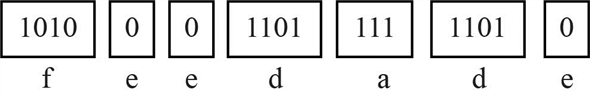The Huffman code for the value "a" is as follows: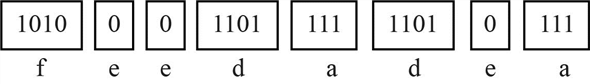The Huffman code for the value "f" is as follows: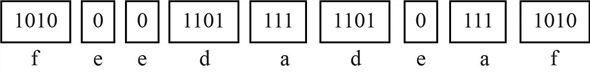The Huffman code for the value "a" is as follows: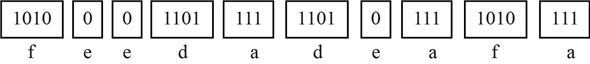The Huffman code for the value "g" is as follows:The Huffman code for the value "e" is as follows: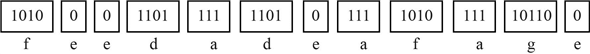The Huffman code for the value "d" is as follows: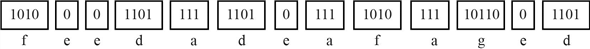The Huffman code for the value "h" is as follows: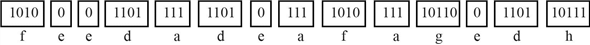The Huffman code for the value "a" is as follows: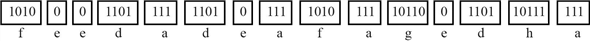The Huffman code for the value "g" is as follows: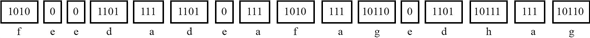Thus the encoded message is as follows: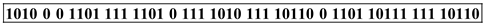Tags
Choose question tagRepeat Exercise 6 for the following table of letters and weights: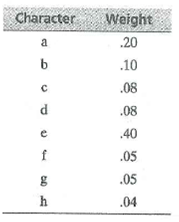Free
Essay

Algorithm for Huffman:
Input:
Characters : [C 1 ,C 2 ,…………C n ]
Weight : [W 1 ,W 2 ,………W n ]
where W i is the weight of C i
Step 1 :
One-node binary tree list is initiated.
Step 2 :
Repeat the process for n-1 times:
• Search for the lowest weight in the list W' and W'' and draw two trees T' and T''
• The trees are replaced with binary tree which contains the root value as W' + W''
• The subtree pointers are labeled as 0 and 1 respectively
Step 3 :
The character's code can be obtained by label in the path from root to the current node
Given:
Use the given table to construct the Huffman code: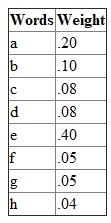As per the Huffman's algorithm, a list of one-node binary tree is constructed in order of increasing order of their weight.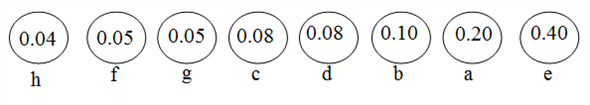The two trees are selected that has the smallest weights; thus it results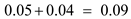.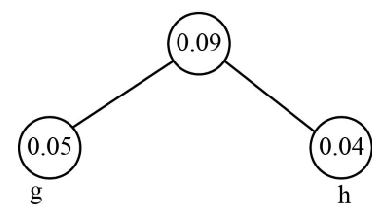From the list, select two of minimal weights "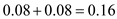".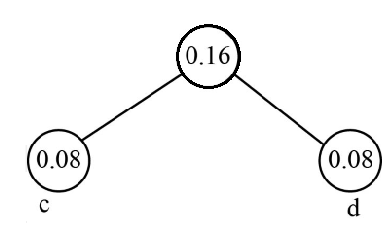From the list of 3 binary trees, select two of minimal weights "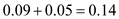".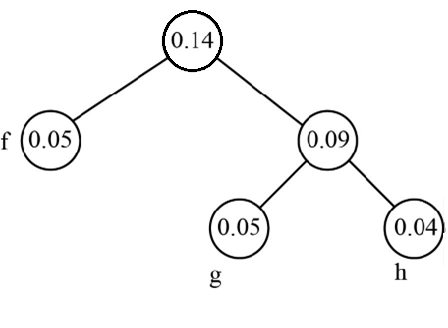From the list, select two of minimal weights "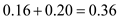":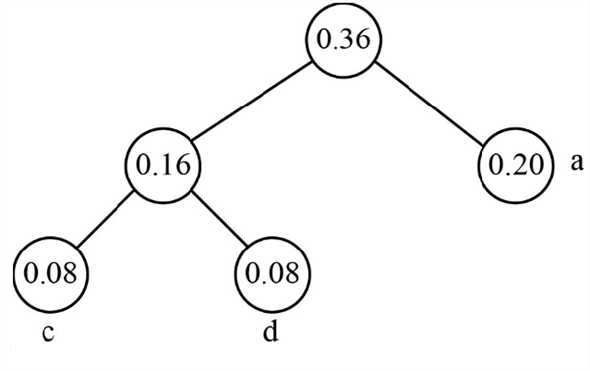From the list, select two of minimal weights "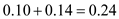".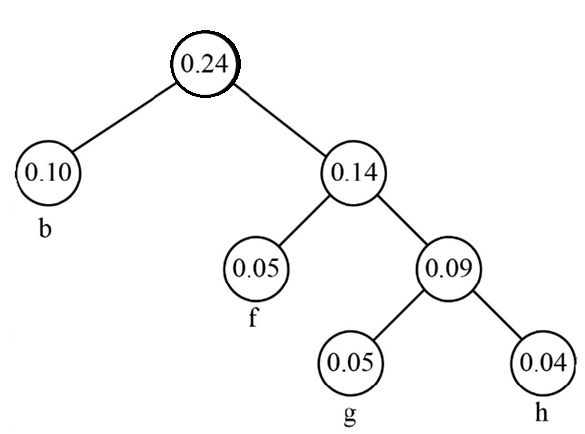Combine the two binary trees to get single resulting Huffman tree: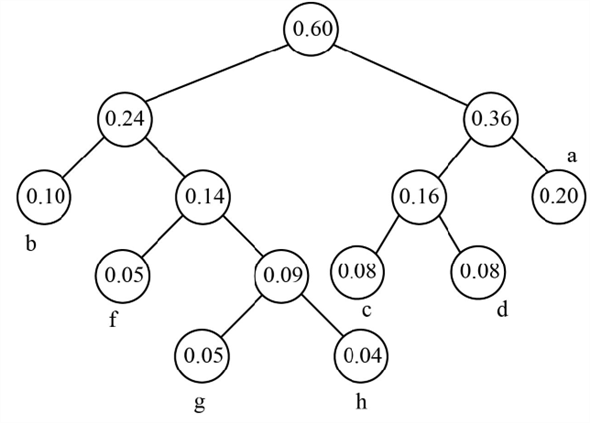Complete Huffman tree.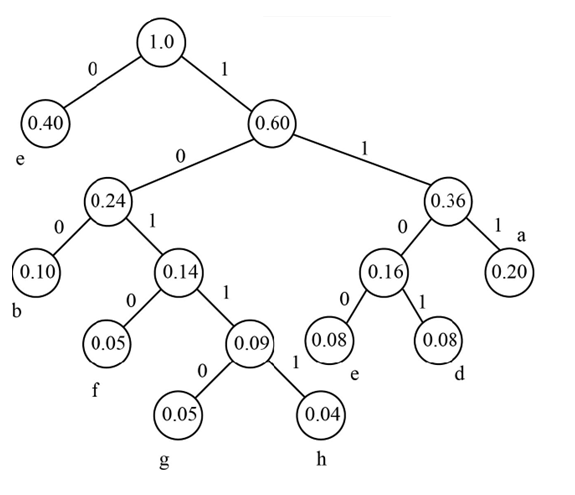Thus the Huffman code obtained from the tree: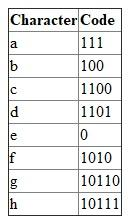Tags
Choose question tagWrite a function that reads a table of letters and their weights and constructs a Huffman code for these letters. Use the function in a program that encodes a message that the user enters.
Free
Essay

Problem plan:
• Include the required header files into the program.
• Use "struct" for tree node
• Allocate new tree to a node
• Define a function "encoding ()" to encode the string.
o check if the root node is null
o check if the leaf node is found
o call the function "encoding ()" to append 0
o call the function "encoding ()" to append 1
• Define a function "huff_tree ()" to generate huffman code
o Use map tp count the frequency
o store live nodes
o create leaf node and push the elements
o perform until more than 1 node in the tree
o pointer is stored to the root of the Huffman
o traverse and store the huffman codes
o print the huffman codes
• Define "main()" to demonstrate huffman technique
o Declare variables
o get input from user
o call the function "huff_tree()" to generate huffman code
Program:
/ ***********************************************************Run the code in c++ 11 compiler *
*Program to construct Huffman code and the encode a *
*message entered by the user *
********************************************************** /
#include
#include
#include
#include
using namespace std;
/ / struct for tree node
struct Node
{
int f;
char ch;
Node *l, *r;
};
/ / allocation of new tree to a node
Node* g_N(char ch, int f, Node* l, Node* r)
{
Node* node = new Node();
/ / assign the node elements
node- ch = ch;
node- f = f;
node- l = l;
node- r = r;
/ / return after all the process has been done
return node;
}
/ / object for comparison
struct comp
{
bool operator()(Node* l, Node* r)
{
/ / the item with highest priority has lowest / / frequency
return l- f r- f;
}
};
/ / traverse and store in an huffman map
void encoding(Node* root, string tx, map huffmanCode)
{
/ / check if the root node is null
if (root == NULL)
return;
/ / if the leaf node is found
if (!root- l !root- r)
huffmanCode[root- ch] = tx;
/ / cal the function to append 0
encoding(root- l, tx + "0", huffmanCode);
/ / call the function to append 1
encoding(root- r, tx + "1", huffmanCode);
}
/ / / build tree and decode
void huff_tree(string inp)
{
/ / to count the frequency
map f;
for (char ch : inp)
f[ch]++;
/ / store live nodes
priority_queue , comp pq;
/ / create leaf node and push the elements
for (auto it : f)
pq.push(g_N(it.first, it.second, NULL, NULL));
/ / perform until more than 1 node in the tree
while (pq.size() != 1)
{
/ / remove nodes with highest priotity
Node *l = pq.top(); pq.pop();
Node *r = pq.top(); pq.pop();
/ / internal node is created and then a new node / / is added
int sum = l- f + r- f;
pq.push(g_N('\0', sum, l, r));
}
/ / pointer is stored to the root of the huffman tree
Node* root = pq.top();
/ / traverse and store the huffman codes
map huffmanCode;
encoding(root, "", huffmanCode);
/ / print the huffman codes
cout "Huffman Code :\n" endl;
for (auto it : huffmanCode)
cout it.first " " it.second endl;
/ / actual string
cout "\n actual string was :\n";
cout inp endl;
/ / print encoded string
string tx = "";
for (char ch : inp)
tx += huffmanCode[ch];
cout "\n encoded string is :\n" tx endl;
}
/ / main function to perform huffman encoding
int main()
{
/ / variable declaration
string inp;
cout "enter the string you want to decode:";
/ / get input from user
cin inp;
/ / call the fuction to generate huffman code
huff_tree(inp);
return 0;
}
Sample Output:
enter the string you want to decode:goodmorning
Huffman Code :
d 1100
g 00
i 1101
m 011
n 111
o 10
r 010
actual string was :
goodmorning
encoded string is :
001010110001110010111110111100

Tags
Choose question tag(Project) Write a program to compress a file using a Huffman code and to decompress a file generated using this code. The program should first read through the file and determine the number of occurrences of each character in the file and the total number of characters in the file. The weight of each character will be the frequency count for that character. The program should then use these weights to construct the Huffman codes for the characters in the file. It should then read the file again and encode it using these Huffman codes and generate a file containing this encoded data. Compute the compression ratio, which is the number of bits in the compressed file divided by the total number of bits in the original file (eight times the number of characters in the file). The program should also provide the option of decompressing a file that was encoded using this Huffman code.
Essay
Tags
Choose question tagFor Exercises 1-5, trace the construction of the AVL tree that results from inserting the C++ keywords in the given order. Show the tree and balance factors for each node before and after each rebalancing. long, const, typedef, bool, public, break, else
Essay
Tags
Choose question tagUsing the first Huffman code given in this section (A = 01, B = 0000, C = 0001, D = 001, E = 1), decode the bit strings in Exercises 2-5. 000001001
Essay
Tags
Choose question tagConstruct the Huffman code for the C++ keywords and weights given in the following table: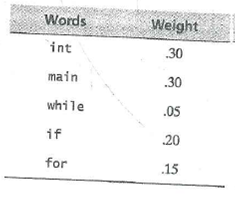Essay
Tags
Choose question tagRepeat Exercise 3 for the following table of C++ keywords and weights (frequencies):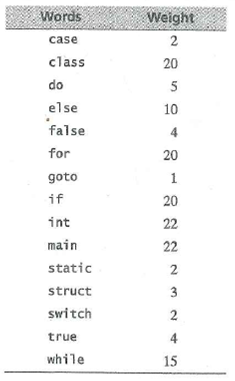Essay
Tags
Choose question tagUsing the first Huffman code given in this section (A = 01, B = 0000, C = 0001, D = 001, E = 1), decode the bit strings in Exercises 2-5. 001101001
Essay
Tags
Choose question tagWrite a driver program to test your general-tree class template from Exercise 26.
Essay
Tags
Choose question tagFor Exercises 1-5, trace the construction of the AVL tree that results from inserting the C++ keywords in the given order. Show the tree and balance factors for each node before and after each rebalancing. unsigned, short, long, int, double, char
Essay
Tags
Choose question tagWrite a driver program to test your red-black tree class template from Exercise 24.
Essay
Tags
Choose question tagUsing the first Huffman code given in this section (A = 01, B = 0000, C = 0001, D = 001, E = 1), decode the bit strings in Exercises 2-5. 00001010011001
Essay
Tags
Choose question tagWrite a driver program to test your B-tree class template from Exercise 25.
Essay
Tags
Choose question tagUse your B-tree class template from Exercise 26 in a program that reads words and constructs a B-tree to store these words. The program should then allow the user to enter a word and should search the B-tree for this word.
Essay
Tags
Choose question tagFor Exercises 1-5, trace the construction of the AVL tree that results from inserting the C++ keywords in the given order. Show the tree and balance factors for each node before and after each rebalancing. do, long, new, and, operator, int, namespace
Essay
Tags
Choose question tagFor Exercises 1-5, trace the construction of the AVL tree that results from inserting the C++ keywords in the given order. Show the tree and balance factors for each node before and after each rebalancing. bool, new, return, struct, while, case, enum
Essay
Tags
Choose question tagUsing the first Huffman code given in this section (A = 01, B = 0000, C = 0001, D = 001, E = 1), decode the bit strings in Exercises 2-5. 000101001
Essay
Tags
Choose question tagDemonstrate that Morse code is not immediately decodable by showing that the bit string 100001100 can be decoded in more than one way.
Essay